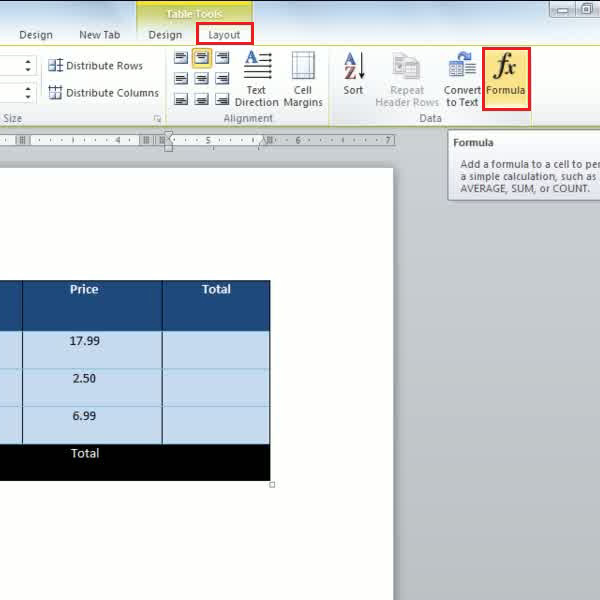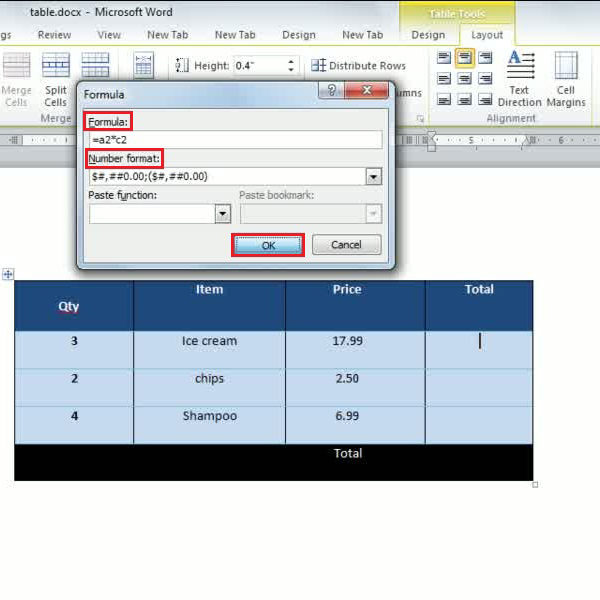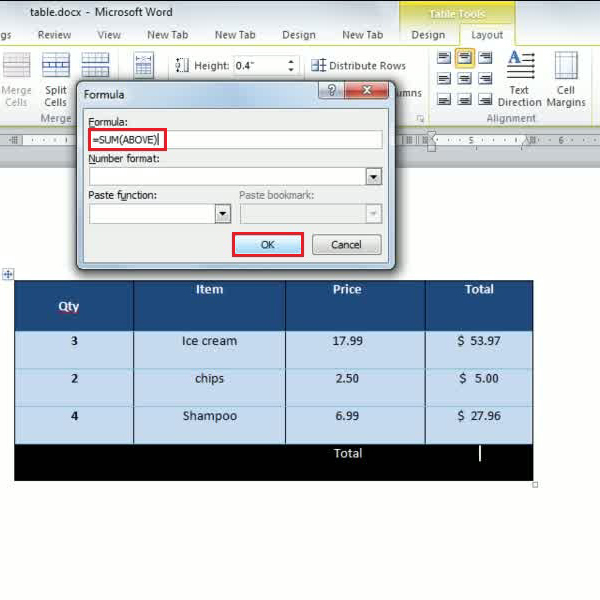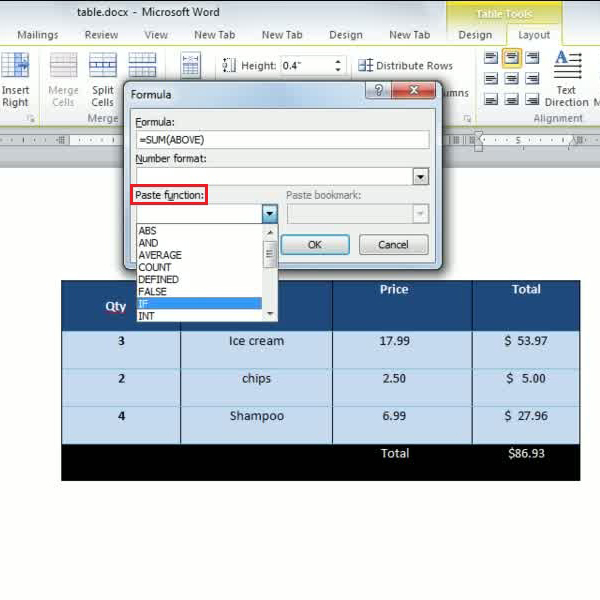## How to Make Calculations in a Table in Microsoft Word 2010

MS word 2010 provides us with a variety of functions and features out of which applying excel functions to word documents is one of the most popular. Mostly, when we use tabular data presentation in our documents, we often need some calculations to be done such as totalling amounts, and calculating the inventory for a month etc. You may not be familiar with the formulas that you can apply to do these calculations, and thus, this office 2010 tutorial will provide you with a step by step guide to help you work out these calculations.
In this tutorial, you will learn how to make calculations in a table in Microsoft Word 2010

Step # 1: Selecting the Column for Results

Before starting any calculation, the first thing you will need to do is select the resultant column. In order to do so, you must first bring your cursor to the cell where you would like to place the calculated value. Now, you must go to the ‘Layout’ tab and click on the option titled ‘Formula’. Here, a new formula window will appear on your screen and with the help of this window, you can apply any formula needed and get the calculations for it in your selected column or cell.Step # 2: Carrying out the Multiplication Process

We will begin with using the multiplication process in tables. In order to multiply a certain quantity with its price, all you need to do is to apply a simple formula. In the previous step, you had opened up a formula window. Now here you must type the formula, ‘=A2*C2’ in the formula field and press the ‘OK’ button to view the result in the selected column. You will also need to select a currency sign that can be attached to the result of the formula applied.
Repeat this process to get the results for 3rd and 4th row by applying the formula “=A3*C3” and “=A4*C4”, respectively.Step # 3 – Using the Sum Operation

After getting the resultant product from the multiplication of Row 1, 2 and 3, if you need to show the sum of all the multiplied values, you will need to click on the last cell of the 4th Row and open the formula window as you had done previously. Once this formula window appears, you must type ‘=SUM (ABOVE)’ and click on the “Ok” button. Now, this will allow you to get the sum of all the values above.Step # 4 – Using the Paste Function

It is often extremely difficult for everyone to remember the exact names and syntax of the various functions provided by the software. In order to help people deal with this problem, MS Word 2010 offers a paste function which contains a list of all available functions in a drop down menu. All you need to do is simply select any function you want to use and the syntax will be directly pasted in the formula field. By using this feature, you can also apply a variety of other functions to your table.